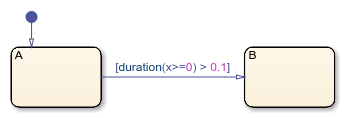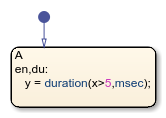# duration

Time during which condition is valid

## Syntax

``time = duration(condition)``
``time = duration(condition,time_unit)``

## Description

example

````time = duration(condition)` returns the length of time, in seconds, that `condition` stays `true` and the associated state became active.```

example

````time = duration(condition,time_unit)` returns the length of time in the unit specified by `time_unit`.```

Note

The temporal logic operator `duration` is not supported in standalone charts in MATLAB®.

## Examples

expand all

Transition out of the state when the variable `x` has been greater than or equal to 0 for longer than 0.1 seconds.

`[duration(x>=0) > 0.1]`Store the number of milliseconds since the variable `x` became greater than 5 and the state became active.

```en,du: y = duration(x>5,msec);```## Input Arguments

expand all

Logical condition, specified as `true` or `false`. You can specify the value of `condition` by using an expression that evaluates to `true` or `false`. The operator evaluates `condition` at each time step.

`condition` does not support expressions that depend on local or output data.

Example: `duration(u)`

Example: `duration(u>=0)`

Units of time that `duration` returns, specified in seconds (`sec`), milliseconds (`msec`), or microseconds (`usec`).

## Tips

• You can use quotation marks to enclose the keywords `'sec'`, `'msec'`, and `'usec'`. For example, `duration(x > 0,'sec')` is equivalent to `duration(x > 0,sec)`.

• The Stateflow® chart resets the value of the `duration` operator if the conditional expression `C` becomes `false` or if the associated state becomes inactive.

• The `duration` operator does not support conditions that depend on local or output structures. For more information, see Access Bus Signals Through Stateflow Structures.

## Version History

Introduced in R2017a